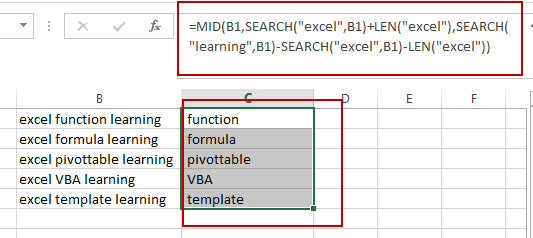# How to Extract Text between Two Text Strings in Excel

This post will guide you how to extract text between two given text strings in Excel. How do I get text string between two words with a formula in Excel.

## Extract Text between Two Text Strings

Assuming that you have a list of data in range B1:B5, in which contain text string values. And you need to extract all text strings between two words “excel” and “learning” in cells. You can use a formula based on the MID function, the SEARCH function, and the Len function to extract text between two specified strings. Like this:

`=MID(B1,SEARCH("excel",B1)+LEN("excel"),SEARCH("learning",B1)-SEARCH("excel",B1)-LEN("excel"))`

Type this formula into a blank cell and press Enter key on your keyboard. And then drag the AutoFill Handle down to other cells to apply this formula.You just need to replace words “excel” and “learning” as you need in the above formula.

You should see that the text string have been extracted between two given works in your data.

### Related Functions

• Excel MID function
The Excel MID function returns a substring from a text string at the position that you specify.The syntax of the MID function is as below:= MID (text, start_num, num_chars)…
• Excel SEARCH function
The Excel SEARCH function returns the number of the starting location of a substring in a text string.The syntax of the SEARCH function is as below:= SEARCH  (find_text, within_text,[start_num])…
• Excel LEN function
The Excel LEN function returns the length of a text string (the number of characters in a text string).The LEN function is a build-in function in Microsoft Excel and it is categorized as a Text Function.The syntax of the LEN function is as below:= LEN(text)…

Sidebar